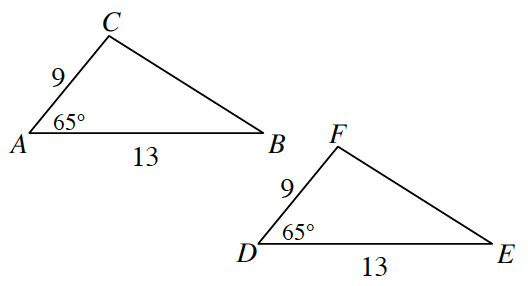### Home > CCG > Chapter 6 > Lesson 6.1.1 > Problem6-5

6-5.Examine the triangles at right.

1. Are these triangles similar? If so, use a flowchart to show how you know. If they are not similar, explain how you know.

Look at the triangles. Do any of the parts make them similar?

They are similar by $\text{SAS}\sim$.

2. Are the triangles congruent? Explain your reasoning.

What is the ratio of the corresponding sides?

Yes, because they are similar and the corresponding sides have a ratio of $1$.